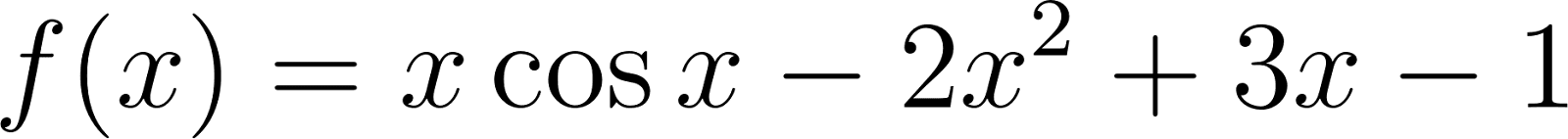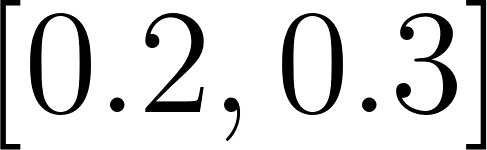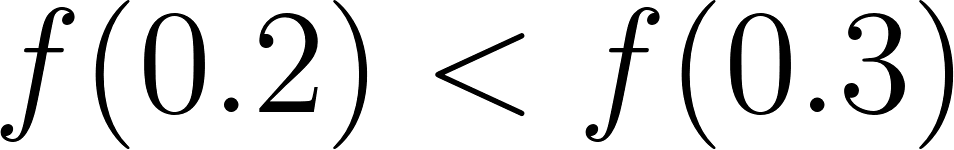×
Get Full Access to Numerical Analysis - 10 Edition - Chapter 1.1 - Problem 1
Get Full Access to Numerical Analysis - 10 Edition - Chapter 1.1 - Problem 1

×

# Answer: Show that the following equations have at least one solution in the givenISBN: 9781305253667 457

## Solution for problem 1 Chapter 1.1

Numerical Analysis | 10th Edition

• Textbook Solutions
• 2901 Step-by-step solutions solved by professors and subject experts
• Get 24/7 help from StudySoup virtual teaching assistantsNumerical Analysis | 10th Edition

4 5 1 251 Reviews
12
4
Problem 1

Show that the following equations have at least one solution in the given intervals. a. a cosa 2a2 + 3a 1 = 0, [0.2, 0.31 and [1.2, 1.3] b. (a-2)2 -InA =0, [1,2] and [e, 4] c. 2a cos(2a) -(a - 2)2 = 0. [2, 3] and [3,4] d. a (In A)r = 0, [4,5]

Step-by-Step Solution:

Problem 1

Show that the following equations have at least one solution in the given intervals.

a. x cos x- 2x2 + 3x -1 = 0, [0.2, 0.3] and [1.2, 1.3]

b. (x-2)2 -In x =0, [1,2] and [e, 4]

c. 2x cos(2x) -(x - 2)2 = 0. [2, 3] and [3,4]

d. x- (In x)x = 0, [4,5].

Step-by-step solution

Step 1 of 16

(a)

(i) Consider the function defined byThe function we will consider when discussing numerical methods will be assumed to be continuous because there is a minimal requirement for predictable behavior.

However, every polynomial function, exponential function, sine function, cosine function and constant function are continuous everywhere.

Therefore,is continuous on.Now,Step 2 of 16

Step 3 of 16

##### ISBN: 9781305253667

Unlock Textbook Solution